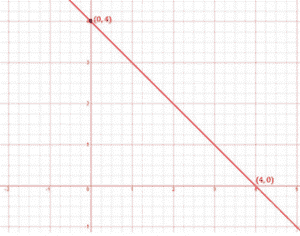# Draw the graph of the following linear equations in two variables: x + y = 4

To draw the graph x+y=4, we have to find the values which x and y can have, satisfying the equation.

We can check that when, x = 0, y = 4,

When x = 0,

x+y = 4

0+y = 4

y = 4

When x = 4,

x+y = 4

4+y = 4

y = 4–4

y = 0

are solutions of the given equation. So, we can use the following table to draw the graph:

 x y 0 4 4 0

The points to be plotted are (0, 4) and (4,0)